# Program to check Spy Number in Java

In this tutorial you will learn about the Program to check Spy Number in Java and its application with practical example.

In this tutorial, we will learn to create a Java Program to check Spy Numbers in Java using Java programming.

## Prerequisites

Before starting with this tutorial we assume that you are best aware of the following Java programming topics:

• Java Operators.
• Basic Input and Output function in Java.
• Class and Object in Java.
• Basic Java programming.
• If-else statements in Java.
• For loop in Java.

## What is Spy Number in Java

A Number is called a Spy number if  sum(1+3+2=6) and product(1*3*6=6) of its digits are equal.

Example:-> Number “22”=> sum(2+2=4) and Product (2*2=4) then “22” is a Spy Number.

## Program to check Spy Number in Java

In this program , we will find given number is a Spy number or not .First of all user will be prompted to enter Number and afterword we will find the given number is a Spy Number or not. Lets have look at the code.

## Output

Spy number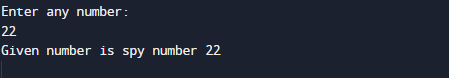Not a Spy number.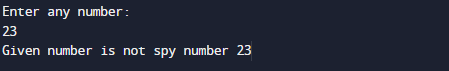In the above program, we have first declared and initialized a set variables required in the program.

• number = it will hold entered number.
• copy = it will hold entered number copy.
• multi  it will hold the result of multiplication of a number.
• Sum=it will hold sum of digit.
• Temp = it will old temp value of number.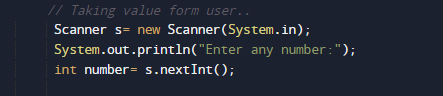## Steps to Find Spy Number

1. take a value from user in (number).
2. take variables sum and product to store value of digit sum and product .
3. Initialize sum with 0 and product with 1.
4. Find the digit (copy%10) of a given number.
5. Add digit to the variable sum=sum+temp;
6. Multiply the digit with the multi= multi*temp;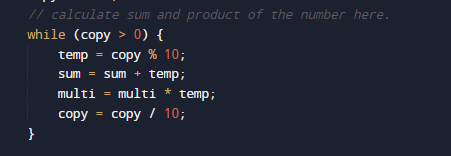1. Divide the number (number) by 10 to removes the last digit.
2. Repeat steps until the number  become 0(Zero).
3. at last check values of sum and product are same, then number is a spy number,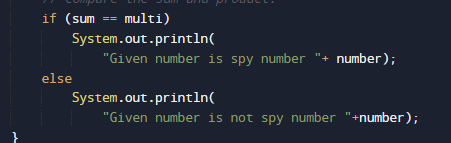1. else not a spy number.

In this tutorial we have learn about the Program to check Spy Number in Java and its application with practical example. I hope you will like this tutorial.